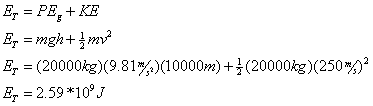# Regents Physics - Conservation of Energy

## Law of Conservation of Energy

"Energy cannot be created or destroyed... it can only be changed."

Chances are you've heard that phrase before. it's one of the most important concepts in all of physics. It doesn't mean that an object can't lose energy or gain energy... what it means is that energy can be changed into different forms, and transferred from system to system, but it never magically disappears or reappears.

Following up on our bank account analogy, if you have a certain amount of money in your bank account, if you spend some money, your bank account balance decreases. Your money wasn't lost, however, it was transferred to another system. It may even change forms... perhaps you purchased an item from another country. Your money is no longer in the form of dollars and cents, but is instead now part of another system in another currency.

There are some issues with our money analogy, however. If the total money supply in a country is low, a government can print more currency. In the world of physics, however, the total amount of energy throughout the universe is fixed... it cannot be replenished. Alternately, governments can collect and destroy currency -- in the world of physics, we can never truly destroy energy. The understanding that the total amount of energy in the universe remains fixed is known as the law of conservation of energy.

## Conservation of Mechanical Energy

As we've learned previously, mechanical energy is the sum of an object's kinetic energy as well as its gravitational potential and elastic potential energies. Non-mechanical energy forms include chemical potential, nuclear, and thermal.

Total energy is always conserved in any system, which is the law of conservation of energy. Confining ourselves to just the mechanical forms of energy, however, if we neglect the effects of friction we can also state that total mechanical energy is constant in any system.Let's take the example of an F/A-18 Hornet jet fighter with a mass of 20,000 kg flying at an altitude of 10,000 meters above the surface of the earth with a velocity of 250 m/s. In this scenario, we can calculate the total mechanical energy of the jet fighter as follows:Now, let's assume the Hornet dives down to an altitude of 2,000 meters above the surface of the Earth. Total mechanical energy remains constant, and the gravitational potential energy of the fighter decreases, therefore the kinetic energy of the fighter must increase. The fighter's velocity goes up as a result of flying closer to the Earth! For this reason, a key concept in successful dogfighting taught to military pilots is that of energy conservation!We can even calculate the new velocity of the fighter jet since we know its new height and its total mechanical energy must remain constant. Solving for velocity at right, we find that the Hornet has almost doubled its speed by "trading in" 8000m of altitude!

If instead we had been told that some of the mechanical energy of the jet was lost to air resistance (friction), we could also account for that by stating that the total mechanical energy of the system is equal to the gravitational potential energy, the kinetic energy, and the change in internal energy of the system (Q). This leads to the conservation of mechanical energy formula given on the Regents Reference Table:## Free Fall Using Energy

Let's take another look at free fall, only this time, let's analyze our falling object using the law of conservation of energy, and compare it to our analysis using the kinematics equations we studied previously.

The problem: An object falls from a height of 10m above the ground. Neglecting air resistance, find its velocity the moment before the object strikes the ground.

### Conservation of Energy Approach

The energy of the object at its highest point must equal the energy of the object at its lowest point, therefore:### Kinematics Approach

For an object in free fall, its initial velocity must be zero, its displacement is 10m, and the acceleration due to gravity on the surface of the Earth is 9.81 m/s2. Choosing down as the positive direction:As you can see, we reach the same conclusion regardless of approach!